Question

# Calculate the number of molecules in (5.47x10^1) grams of sulfur trioxide, SO3. Provide your answer to...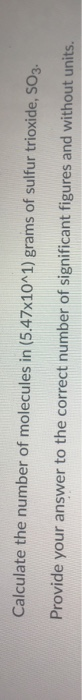Calculate the number of molecules in (5.47x10^1) grams of sulfur trioxide, SO3. Provide your answer to the correct number of significant figures and without units.

Molecular mass of SO3 = 80.066g /mol

Now for (5.47 × 10 ^1)g of SO3 number of moles = 54.7g/80.066g/mol

= 0.683mol

Thus number of molecule = number of moles × Avogadro Number

= 0.683 × 6.022 × 1023

= 4.11 × 1023 molecules

#### Earn Coins

Coins can be redeemed for fabulous gifts.

Similar Homework Help Questions
• ### A sample of sulfur trioxide (SO3) weighs 5100g. How many molecules are contained in this sample?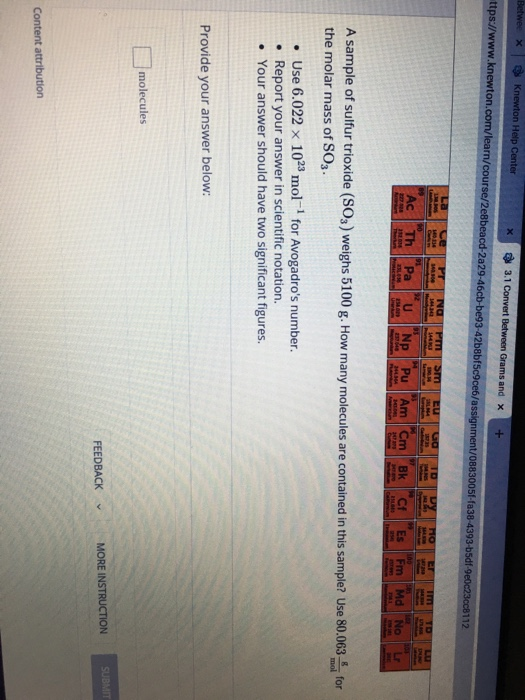A sample of sulfur trioxide (SO3) weighs 5100g. How many molecules are contained in this sample? Use 80.063 the molar mass of SO3. Use 6.022 x 1023 mol-1 for Avogadro's number. Report your answer in scientific notation.Your answer should have two significant figures.

• ### Sulfur dioxide reacts with carbon dioxide to form an equilibrium with sulfur trioxide and carbon monoxide, all gases (see the reaction below).

Sulfur dioxide reacts with carbon dioxide to form an equilibrium with sulfur trioxide and carbon monoxide, all gases (see the reaction below). A mixture of 1.81 mole each of sulfur dioxide and carbon dioxide is placed in an 2.1 L container and allowed to reach equilibrium. If the equilibrium constant, Kc, is 1.21 at this temperature, what is the concentration of sulfur trioxide at equilibrium? SO2 (g) + CO2 (g) ⇌ SO3 (2)+ CO (g) Keep extra significant figures during the calculation and...

• ### Question 5 (1 point) Sulfur trioxide gas can be synthesized by the reaction of sulfur dioxide...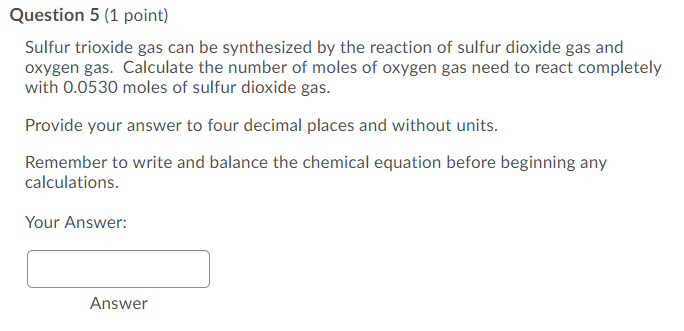Question 5 (1 point) Sulfur trioxide gas can be synthesized by the reaction of sulfur dioxide gas and oxygen gas. Calculate the number of moles of oxygen gas need to react completely with 0.0530 moles of sulfur dioxide gas. Provide your answer to four decimal places and without units. Remember to write and balance the chemical equation before beginning any calculations. Your Answer: Answer

• ### 1.) Acid rain forms in the upper atmosphere by the reaction of sulfur trioxide with water...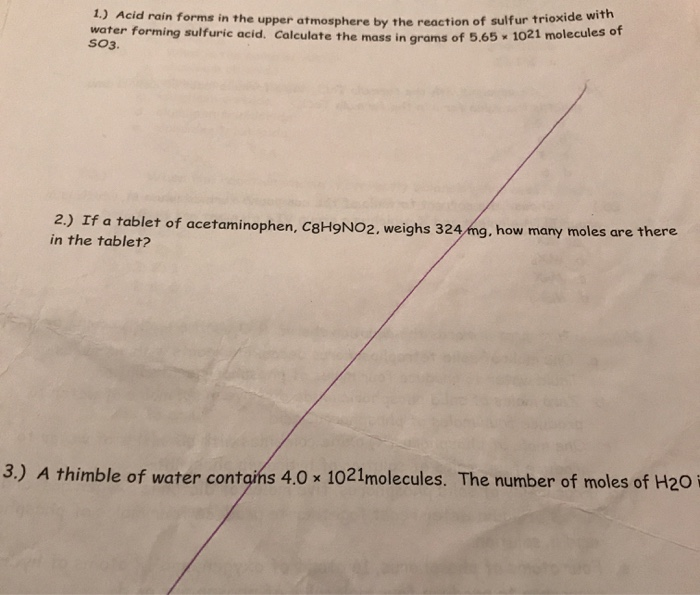1.) Acid rain forms in the upper atmosphere by the reaction of sulfur trioxide with water forming sulfuric acid. Calculate the mass in grams of 5.65 1021 molecules of SO3. 2.) If a tablet of acetaminophen, C8H9NO2, weighs 324 mg, how many moles are there in the tablet? 3.) A thimble of water contains 4.0 x 1021molecules. The number of moles of H20 i

• ### Question 2 (2 points) Calculate the mass of (3.28x10^25) atoms of nickel metal. Provide your answer...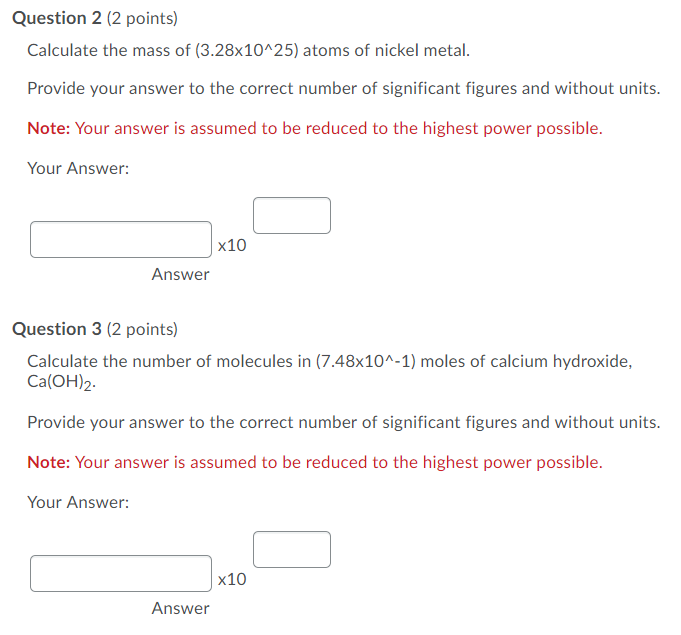Question 2 (2 points) Calculate the mass of (3.28x10^25) atoms of nickel metal. Provide your answer to the correct number of significant figures and without units. Note: Your answer is assumed to be reduced to the highest power possible. Your Answer: X10 Answer Question 3 (2 points) Calculate the number of molecules in (7.48x10^-1) moles of calcium hydroxide, Ca(OH)2 Provide your answer to the correct number of significant figures and without units. Note: Your answer is assumed to be reduced...

• ### determine the number of resonance structures for nitrate ion, NO3- and Sulfur trioxide molecule SO3-. please...

determine the number of resonance structures for nitrate ion, NO3- and Sulfur trioxide molecule SO3-. please explain?

• ### Calculate the change in the standard molar entropy of sulfur trioxide gas, SO3, when it is...

Calculate the change in the standard molar entropy of sulfur trioxide gas, SO3, when it is cooled from a temperature of 100°C to 10°C at a constant pressure of 1 bar. *use equation ∆S = nCpln(T2/T1)

• ### Sulfur dioxide and oxygen react to form sulfur trioxide, like this: 2SO2(g)+O2(g)→2SO3(g) Also, a chemist finds...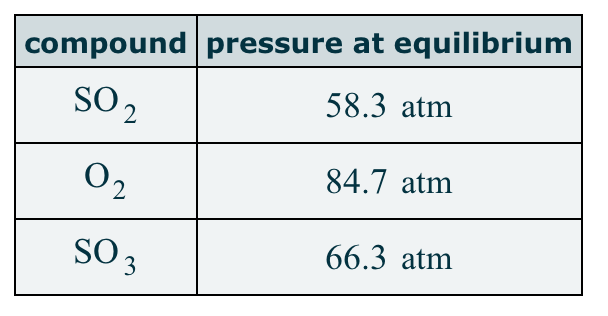Sulfur dioxide and oxygen react to form sulfur trioxide, like this: 2SO2(g)+O2(g)→2SO3(g) Also, a chemist finds that at a certain temperature the equilibrium mixture of sulfur dioxide, oxygen, and sulfur trioxide has the following composition: Calculate the value of the equilibrium constant Kp for this reaction. Round your answer to 2 significant digits. compound pressure at equilibrium SO2 58.3 atm 02 84.7 atm SO3 66.3 atm

• ### Sulfur dioxide and oxygen react to form sulfur trioxide during one of the key steps in...

Sulfur dioxide and oxygen react to form sulfur trioxide during one of the key steps in sulfuric acid synthesis. An industrial chemist studying this reaction fills a 25.0L tank with 8.8 mol of sulfur dioxide gas and 2.4 mol of oxygen gas, and when the mixture has come to equilibrium measures the amount of sulfur trioxide gas to be 0.48 mol. Calculate the concentration equilibrium constant for the reaction of sulfur dioxide and oxygen at the final temperature of the...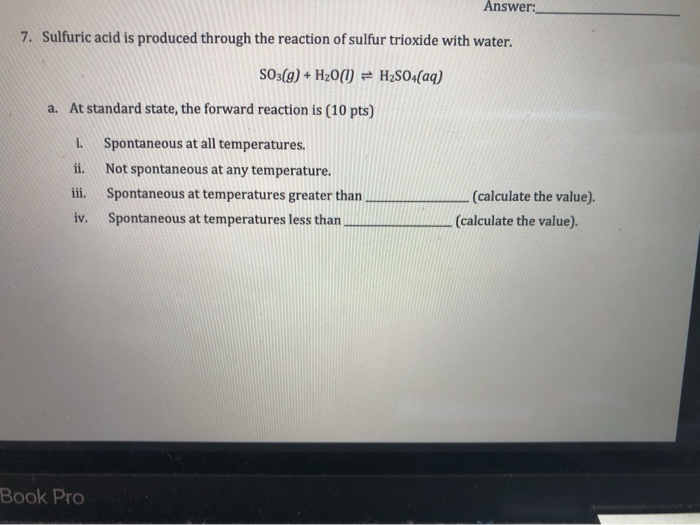please help! Answer: 7. Sulfuric acid is produced through the reaction of sulfur trioxide with water. SO3(g) + H200 = H2SO4(aq) a. At standard state, the forward reaction is (10 pts) Spontaneous at all temperatures. ii. Not spontaneous at any temperature. iii. Spontaneous at temperatures greater than iv. Spontaneous at temperatures less than (calculate the value) (calculate the value). Book Pro b. 0.801 g sulfur trioxide is added to 1.00 L of water at 298 K. Calculate the equilibrium concentration...